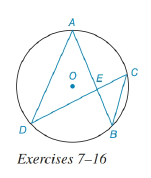Chapter 6.3, Problem 14EElementary Geometry For College St...

7th Edition
Alexander + 2 others
ISBN: 9781337614085

Solutions

Chapter
SectionElementary Geometry For College St...

7th Edition
Alexander + 2 others
ISBN: 9781337614085
Textbook Problem

In the figure for Exercises 7 to 16, O is the center of the circle. See Theorem 6.3.5.Given: A E = x 2 , E B = x 3 , D E = 5 x 6 ,     a n d     E C = 6 Find: x and DE.To determine

To find:

To find x and DE

Explanation

Given that, AE=x2,EB=x3,DE=5x6,andEC=6

The diagrammatic representation is given below,

Theorem:

If two chords intersect within a circle, then the product of the lengths of the segments (parts) of one chord is equal to the product of the lengths of the segments of the other chord.

By using the theorem to get the following,

AEEB=DEEC

Substitute the values AE=x2,EB=x3,DE=5x6,andEC=6 in the above equation to get the following,

Still sussing out bartleby?

Check out a sample textbook solution.

See a sample solution

The Solution to Your Study Problems

Bartleby provides explanations to thousands of textbook problems written by our experts, many with advanced degrees!

Get Started

Convert the expressions in Exercises 31-36 to positive exponent form. 34x2/3

Finite Mathematics and Applied Calculus (MindTap Course List)

Solve each inequality for x. 55. (a) ln x 0 (b) ex 5

Single Variable Calculus: Early Transcendentals, Volume I

Subtract: 4002.81

Elementary Technical Mathematics

For y = sin 6x, dydx=. a) cos 6x b) 6 cos x c) cos 6 d) 6 cos 6x

Study Guide for Stewart's Single Variable Calculus: Early Transcendentals, 8th

In Exercises 1-18, construct a truth table for each compound proposition. p(qr)

Finite Mathematics for the Managerial, Life, and Social Sciences